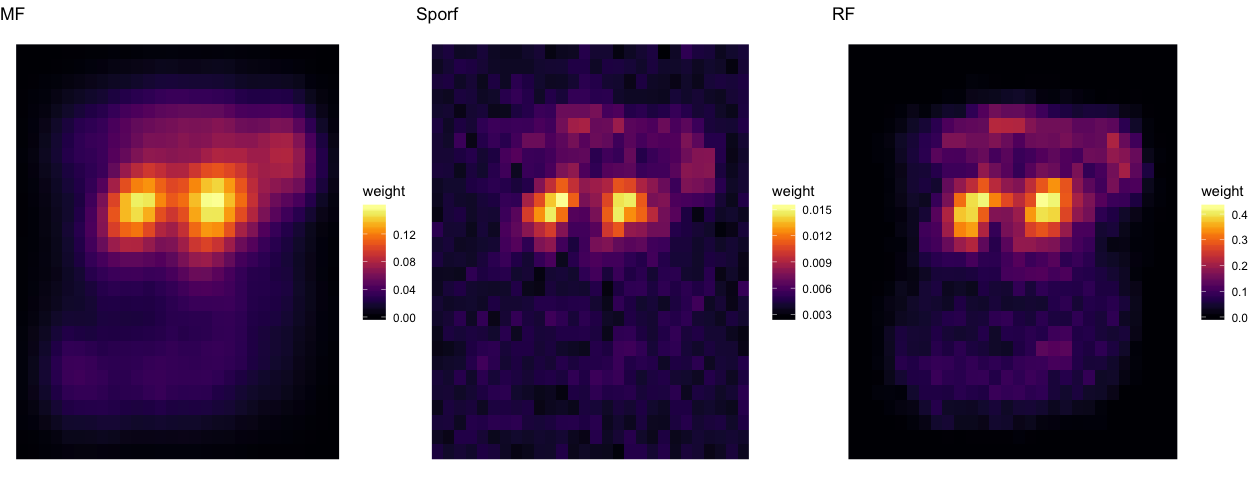# Feature Importance on MNIST with feature counts¶

## Select a subset of 3’s and 5’s from MNIST¶

Here we train a forest using RerF with the RandMatImagePatch option with patch.min = 1 and patch.max = 5.

## Get a random subsample, 100 each of 3's and 5's
threes <- which(mnist$Ytrain %in% 3) fives <- which(mnist$Ytrain %in% 5)
numsub <- c(threes, fives)

Ytrain <- mnist$Ytrain[numsub] Xtrain <- mnist$Xtrain[numsub,]
Ytest <- mnist$Ytest[mnist$Ytest %in% c(3,5)]
Xtest <- mnist$Xtest[mnist$Ytest %in% c(3,5),]

# p is number of dimensions, d is the number of random features to evaluate, iw is image width, ih is image height, patch.min is min width of square patch to sample pixels from, and patch.max is the max width of square patch
p <- ncol(Xtrain)
d <- ceiling(sqrt(p))
iw <- sqrt(p)
ih <- iw
patch.min <- 1L
patch.max <- 5L

forestS <- RerF(Xtrain, Ytrain, num.cores = numCores, FUN = RandMatImagePatch,
paramList = list(p = p, d = d, iw = iw, ih = ih,
pwMin = patch.min, pwMax = patch.max), max.depth = 8)

predS <- Predict(Xtest, forestS, num.cores = numCores)
(mnist.error.rate <- mean(predS != Ytest))

##  0.01892744

forestRerF <- RerF(Xtrain, Ytrain, num.cores = numCores, FUN = RandMatBinary,
paramList = list(p = p, d = d), max.depth = 8)

predRerF <- Predict(Xtest, forestRerF, num.cores = numCores)
(mnist.error.rate <- mean(predRerF != Ytest))

##  0.02208202

forestRF <- RerF(Xtrain, Ytrain, num.cores = numCores, FUN = RandMatRF,
paramList = list(p = p, d = d), max.depth = 8)

predRF <- Predict(Xtest, forestRF, num.cores = numCores)
(mnist.error.rate <- mean(predRF != Ytest))

##  0.02050473

system.time({
FeatImpS <- FeatureImportance(forestS, num.cores = numCores, type = "C")
})

## Message: Computing feature importance as counts of unique feature combinations.

##     user   system  elapsed
## 1229.062    6.560  314.241

ni <- matrix(0, length(FeatImpS$features), ncol(Xtrain)) for(i in 1:length(FeatImpS$features)){
ni[i, FeatImpS$features[[i]]] <- FeatImpS$imp[i]
}

NN <- matrix(apply(ni, 2, sum) / nrow(ni), 28, 28, byrow = FALSE)
saveRDS(NN, file = "NNsrerf.rds")

system.time({
FeatImpRerF <- FeatureImportance(forestRerF, num.cores = numCores, type = "C")
})

## Message: Computing feature importance as counts of unique feature combinations.

##     user   system  elapsed
## 1750.585   11.082  444.860

nir <- matrix(0, length(FeatImpRerF$features), ncol(Xtrain)) for(i in 1:length(FeatImpRerF$features)){
nir[i, FeatImpRerF$features[[i]]] <- FeatImpRerF$imp[i]
}

NNr <- matrix(apply(nir, 2, sum) / nrow(nir), 28, 28, byrow = FALSE)
saveRDS(NNr, file = "NNrerf.rds")

system.time({
FeatImpRF <- FeatureImportance(forestRF, num.cores = numCores, type = "C")
})

## Message: Computing feature importance as counts of unique feature combinations.

##    user  system elapsed
##  14.579   0.323   5.549

nirf <- matrix(0, length(FeatImpRF$features), ncol(Xtrain)) for(i in 1:length(FeatImpRF$features)){
nirf[i, FeatImpRF$features[[i]]] <- FeatImpRF$imp[i]
}

NNrf <- matrix(apply(nirf, 2, sum) / nrow(nirf), 28, 28, byrow = FALSE)
saveRDS(NNrf, file = "NNrf.rds")


## plot the average 3 and 5 from the training set¶

the3s <- Xtrain[Ytrain == 3, ]
the5s <- Xtrain[Ytrain == 5, ]

sum3 <- matrix(apply(the3s, 2, sum) / sum(Ytrain == 3), 28, 28, byrow = FALSE)
sum5 <- matrix(apply(the5s, 2, sum) / sum(Ytrain == 5), 28, 28, byrow = FALSE)

g <- expand.grid(x = 1:28, y = 1:28)
gg <- rbind(g, g)

nn <- as.vector(t(NN[28:1, ]))
nnrerf <- as.vector(t(NNr[28:1, ]))
nnrf <- as.vector(t(NNrf[28:1, ]))

s3 <- as.vector(t(sum3[28:1,]))
s5 <- as.vector(t(sum5[28:1,]))
s3m5 <- abs(s3 - s5)

Z <- data.frame(g, weight = c(nn, nnrerf, nnrf, s3, s5, s3m5), Alg =  rep(c("MF", "Sporf", "RF", "Average 3", "Average 5", "x3m5"), each = length(nn)))

a1 <- ggplot(data = Z[ Z$Alg == "Average 3", ], aes(x = x, y = y, fill = weight)) + geom_raster() + theme_void() + guides(fill = FALSE) + sc1 + ggtitle("Average 3") a2 <- ggplot(data = Z[ Z$Alg == "Average 5", ], aes(x = x, y = y, fill = weight)) + geom_raster() + theme_void() + guides(fill = FALSE) + sc1 + ggtitle("Average 5")
a3 <- ggplot(data = Z[ Z$Alg == "x3m5", ], aes(x = x, y = y, fill = weight)) + geom_raster() + theme_void() + guides(fill = FALSE) + sc1 + ggtitle("abs(avg(3) - avg(5))") grid.arrange(a1, a2, a3, ncol=3)#ggslackr(grid.arrange(a1, a2, a3, ncol=3), channels="#manifold-forest")  ## Feature heatmap¶ These are the features that S-RerF and RerF used, plotted as averaged heatmaps. p1 <- ggplot(data = Z[ Z$Alg == "MF",    ], aes(x = x, y = y, fill = weight)) + geom_raster() + theme_void() + sc1 + ggtitle("MF")
p2 <- ggplot(data = Z[ Z$Alg == "Sporf", ], aes(x = x, y = y, fill = weight)) + geom_raster() + theme_void() + sc1 + ggtitle("Sporf") p3 <- ggplot(data = Z[ Z$Alg == "RF",    ], aes(x = x, y = y, fill = weight)) + geom_raster() + theme_void() + sc1 + ggtitle("RF")